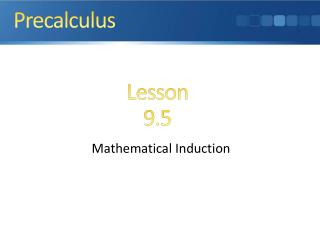# Mathematical Induction - PowerPoint PPT PresentationDownload PresentationMathematical Induction

Mathematical Induction
Download Presentation## Mathematical Induction

- - - - - - - - - - - - - - - - - - - - - - - - - - - E N D - - - - - - - - - - - - - - - - - - - - - - - - - - -
##### Presentation Transcript

1. Precalculus Lesson 9.5 Mathematical Induction

2. Quick Review

3. What you’ll learn about • The Tower of Hanoi Problem • Principle of Mathematical Induction • Induction and Deduction … and why The principle of mathematical induction is a valuable technique for proving combinatorial formulas.

4. Back to our sequence Look at the factors. 1•6 1•12 1•20 1•30 1•42 1•56 2•3 2•6 2•10 2•15 2•21 2•28 3•4 4•5 3•10 3•14 3•12 4•13 4•14 5•6 6•7 7•8 Notice any patterns in the factors?

5. Compare factors to n ? 462 2•3 3•4 4•5 5•6 6•7 7•8 How does the 1st factor relate to n ? Isn’t each one more than the number (n)? Isn’t each two more than the number (n)? (n + 1) (n + 2) How about the 2nd factors and n ?

6. Example: Use the figures and inductive reasoning to complete the table then find the nth term. Look at the factors 1•2 1•5 1•9 1•14 1•20 1•27 3•3 2•7 2•10 3•9 4•5 Notice any patterns in the factors? What if we double the figurate numbers?

7. Example: Doubled 230 4 10 18 28 40 54 (n)(n+3) 460 (n)(?) 1•4 1•10 1•18 1•28 1•40 1•54 Now lets look at the factors. 2•2 2•5 2•9 2•14 2•20 2•27 3•6 4•7 5•8 3•18 6•9 How do the 1st factors relate to n? How do the 2nd factors relate to n? So if we doubled it to get an answer, then we must take half of the answer.

8. Principle of Mathematical Induction Let Pn be a statement about the integer n. Then Pn is true for all positive integers n provided the following conditions are satisfied: • (the anchor) P1 is true; • (inductive step) if Pk is true, • then Pk+1 is true.

9. Example Proof by Induction True and

10. Example Proof by Induction True Factoring out the common factor of (k+1)(k+2)

11. Example Proving Divisibility Which is divisible by 3. Since both terms are divisible by 3, so is the sum, so Pk+1 is true. Therefore, Pn is true for all n ≥ 1.

12. Homework: Text pg756 (pg755 old edition) Exercises # 2-20 even